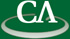## Rational number reconstruction.

### Michael Monagan, Department of Mathematics, Simon Fraser University.

Wednesday July 18, 2007, K9509, 2:30-4:30pm.

```Abstract:
Suppose we have computed a rational number n/d modulo some integer m.
The problem of rational reconstruction is, given u = n/d mod m, and m,
find n/d.  We describe two algorithms for doing this.
Both require that m is larger than 2|n|d.  Both are based on the Euclidean
algorithm and have complexity O(N^2) where N = log m.

We apply rational number reconstruction to solve a linear system
Ax=b over the rationals by first solving Ax=b mod p for some prime p,
then using linear p-adic lifting to solve Ax=b mod p^k then
recovering the solution vector x using rational reconstruction modulo p^k.
We also describe an algorithm which uses Cramer's rule and
Chinese remaindering to solve Ax=b.

```